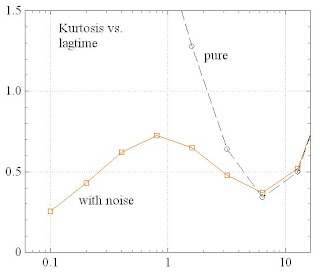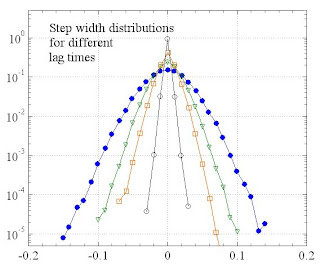## Wednesday, April 23, 2008

### [AD 12] Results for the SD model

The SD model in two (independent) spatial dimensions has been implemented and it seems to work.

According to the SD model (see [AD 11]), the autocorrelation should have a powerlaw distribution with some prescribed exponent p. This will produce long persistent chains of the same sign/direction. The length distribution of the chains will be a PL with another exponent gamma. The analytical relation between p and gamma is not known yet. In the simulation, it is actually the exponent gamma which is prescribed.

For the step length d, a Poisson distribution was chosen. Parameters are the width wid1 of a single step and the average time tauAv between successive steps.

Another parameter is the variance sig2Noise of the additive white Gaussian noise.

For the choice:

wid1=1.0e-2;
tauAv=1.5;
gamma=-2.5;
sig2Noise=0.25e-4;

and a maximum chain length of 1e4, the MSD looks as expected:Also the kurtosis behaves as in the experiments:The step width distributions (without noise) show the characteristic exponential-like form for small lag times:It is interesting that the "fit" parameters indicate force steps with an individual magnitude of 10 nm, following in average intervalls of 1.5 sec. This should be intrepreted biologically.